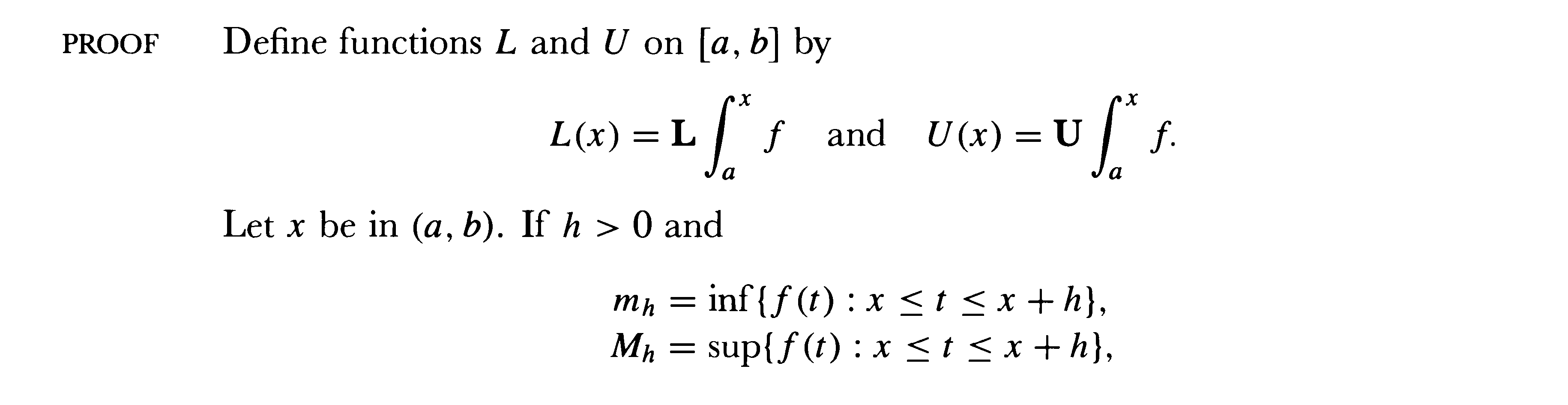Math 316 Notes and Summaries This page requires Mozilla/Firefox/Netscape 7+ or Internet Explorer 6+ MathPlayer .

Martin Flashman's Courses
MATH 316 Real Analysis I Spring, 2016
Class Notes and Summaries
Week 10 3-21, 3-22, 3-24, 3-25, 2016

3-21 Listed Main properties of the definite integral that we will prove:
Included: Monotonicity. Linearity. Additivity. Bounded Constraint. Continuity of Integral Function for integrable functions. Continuous Functions are integrable. Fundamental Theorem (Derivative form) for Continuous Functions. FTofC (Evaluation form) for Continuous Functions. Mean Value Theorem for Integrals for Continuous Functions.

3-22.

Recall the definition of the Darboux Integral for f a bounded function on [a,b].

Suppose P =\{ a=x_0<x_1<...<x_{n-1}<x_n=b\}; I_k = [x_{k-1},x_k]; Delta x_k=x_k-x_{k-1} for k=1,2,...,n.
M_k = lub\{f(x): x in I_k\};  m_k= glb\{f(x): x in I_k\}
 U(P)=\sum_{k=1}^n M_k cdot Delta x_k ;L(P)=\sum_{k=1}^n m_k cdot Delta x_k ; and
int_U f= glb\{U(P)\}; \int_L f= glb\{L(P)\}.
Then f is (Darboux) integrable over [a,b] if \int _U f =\int_L f , which is then denoted \int_a^b f.
Note: We have shown that f is integrable if and only if for any epsilon > 0 there is a partition P where U(P)-L(P) < epsilon.
Also
If f is Darboux integrable then f is Riemann integrable. [For any partition, L(P) \le R(P,C) \le U(P) ]

Linearity and Monotonicity. [Proof omitted here.]
i. If f and g are integrable over [a,b] and alpha in R then alpha f + g is integrable over [a,b] and
\int_a^b  alpha f  + g = alpha \int_a^b f + \int_a^b g.
ii.a If f(x) \ge 0  for all x in [a,b] and f is integrable then  \int_a^b f \ge 0.

ii.b If f(x) \ge g(x)  for all x in [a,b] and f and g are integrable then  \int_a^b f \ge \int_a^b g.

Additivity. Continuity of Integral Function for Integrable Functions. Continuous Functions are Integrable.

Excerpt from Spivak, Calculus, Ch 13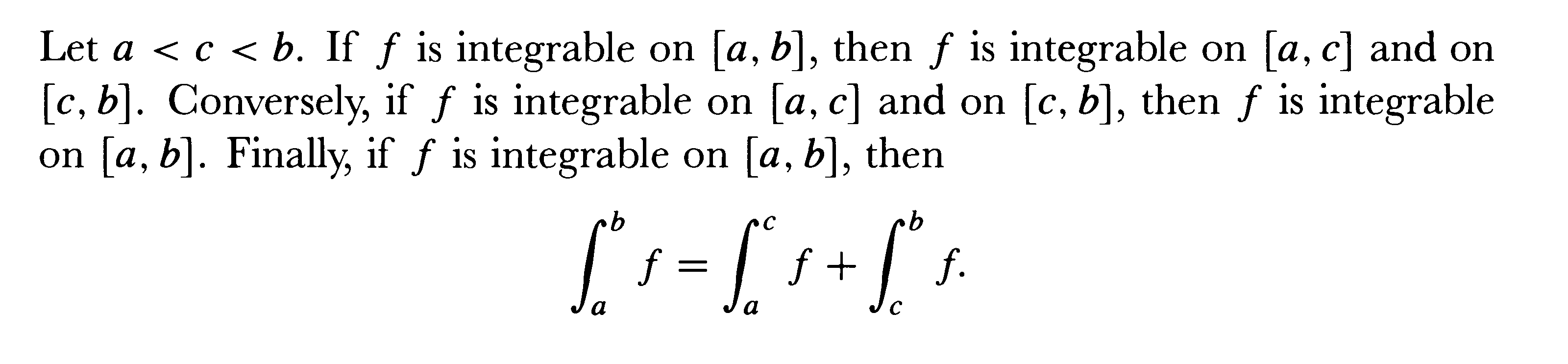Proof: [Converse proof omitted]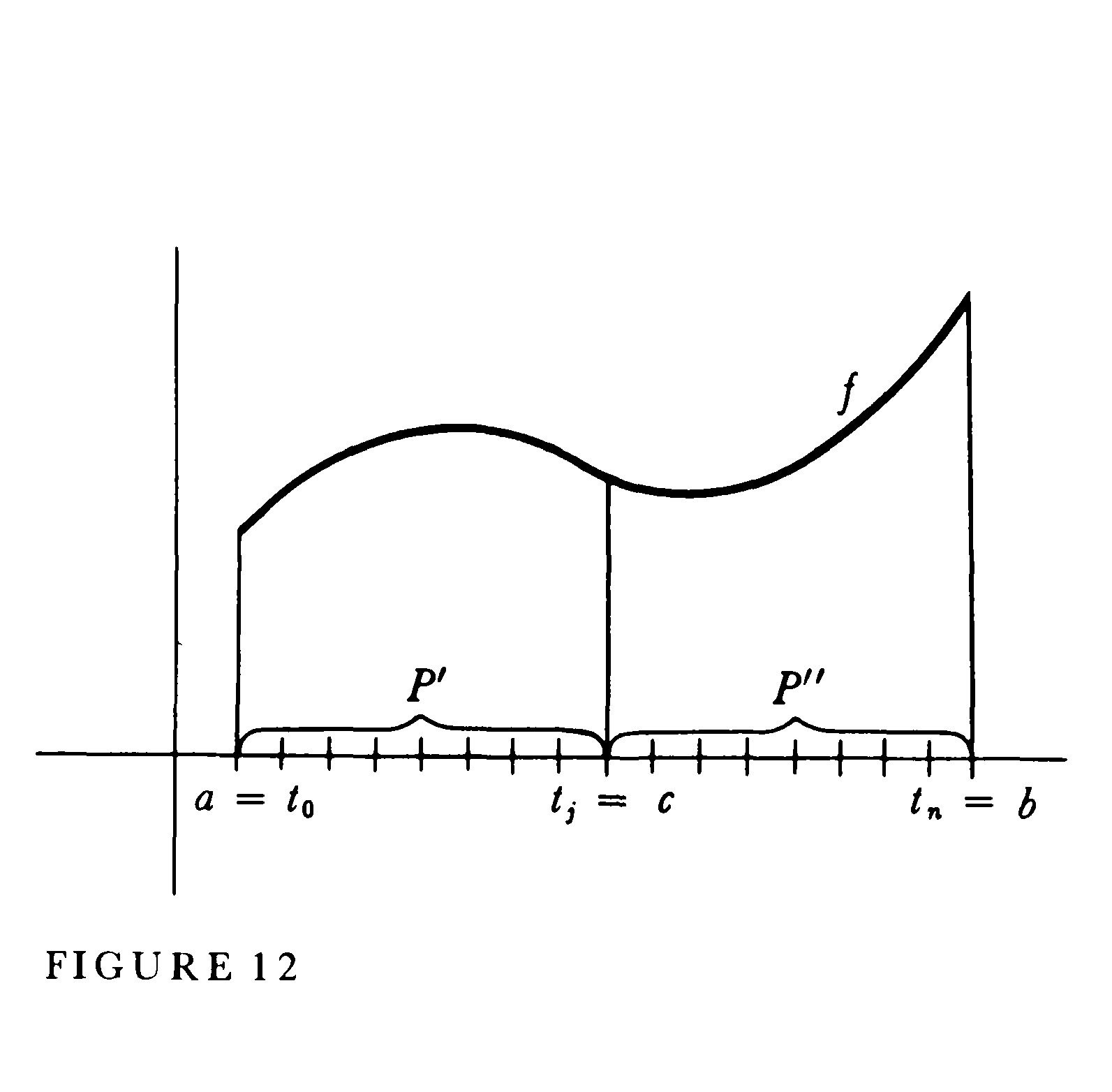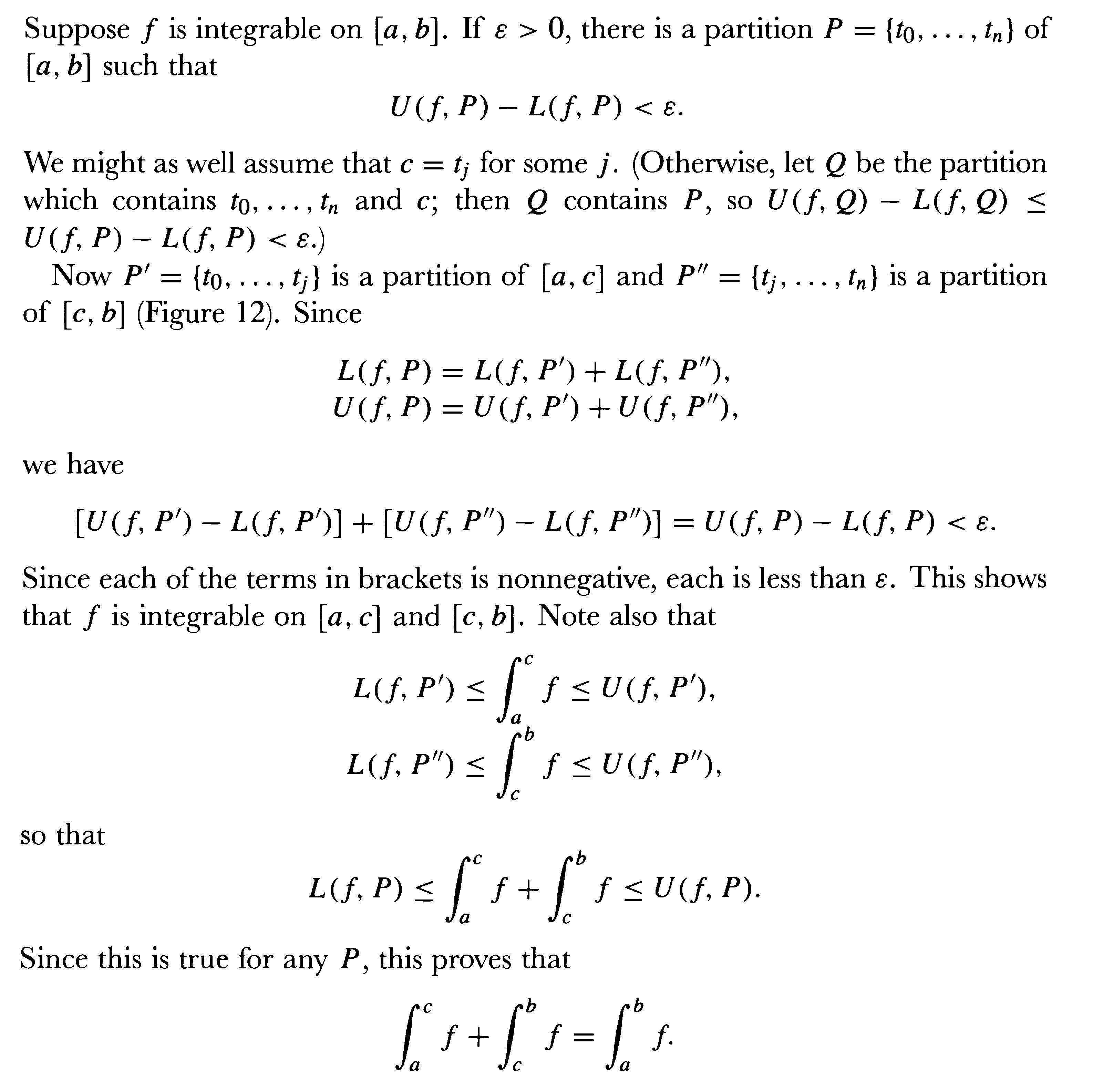EOP

Theorem:[Boundedness]  Suppose f  is integrable on [a,b] and there exist m and M so that for all x in [a,b] m \le f(x) \le M. Then m(b-a) \le \int_a^b f \le M(b-a).

Proof: Consider any partition P of [a,b], then m(b-a) \le L(f,P) and U(f,P) \le M(b-a).
Now since f is integrable,
m(b-a) \le L(f,P) \le lub {L(f,P)} =  \int_a^b f = glb{U(f,P)} \le U(f,P) \le M(b-a).  EOP

Excerpted from Spivak, Calculus, Chapter 13:

Theorem: If f  is bounded and integrable on [a,b] and  F(x) = \int_a^x f  for x in [a,b] then F is continuous on [a,b].

Proof:
Since f is bounded on the interval [a,b], we have a number M >0 so that for all x in [a,b],  -M \le f(x) \le M.
Now consider F on the interval [c, c+h], of length h>0.
Then
F(c+h) = \int_a^{c+h} f  and using additivity we have F(c+h) - F(c) = \int_c^{c+h} f .
By the previous result we have
-Mh \le  \int_c^{c+h}f  \le Mh  or
(1)    -Mh \le  F(c+h) - F(c)  \le Mh

Now consider F on the interval [c+h, c], where h<0, and length -h>0.
Then using the additive property we have F(c) = \int _a^c f = \int _a^{c+h} f + \int_{c+h}^c f and so
F(c+h)-F(c) = -\int_{c+h}^c f.
Using boundedness we have then that
-M(-h)  \le \int_{c+h}^c f  \le M(-h) or M(-h)  \ge -\int_{c+h}^c f  \ge M(h).
Thus we have when h <0 that Mh \le F(c+h) - F(c) \le M(-h). In either case we see that as h to 0 the squeeze theorem can apply , showing that F(c+h)-F(c) to 0  or lim_{x to c} f(x) = f(c), and thus F is continuous at x=c for all c in [a,b].   EOP

3-24. FTof Calc I and II, MVT for Integrals. Alternative proof of FTofC using MVT for Integrals.

Excerpted from Spivak, Calculus, Chapter 14: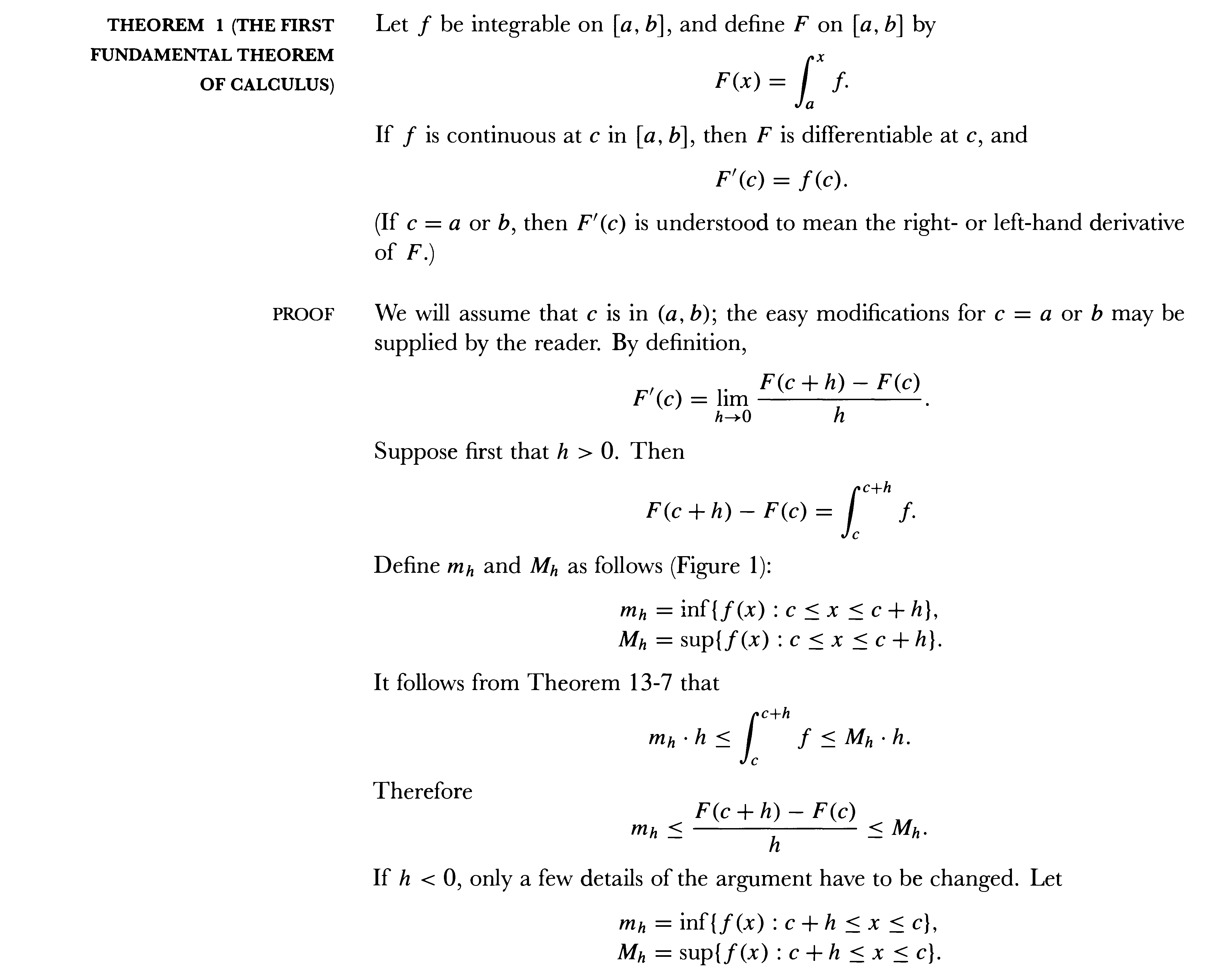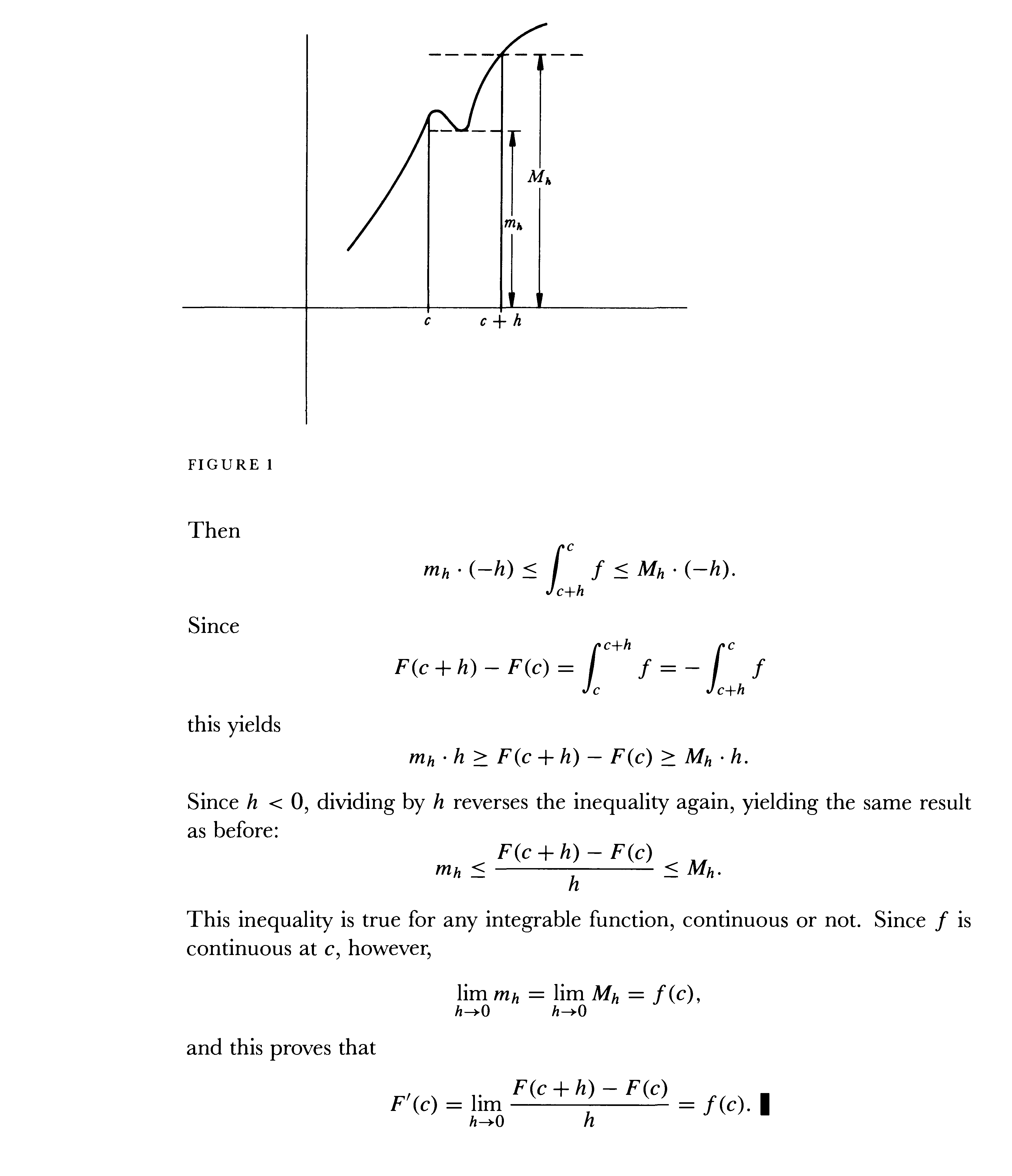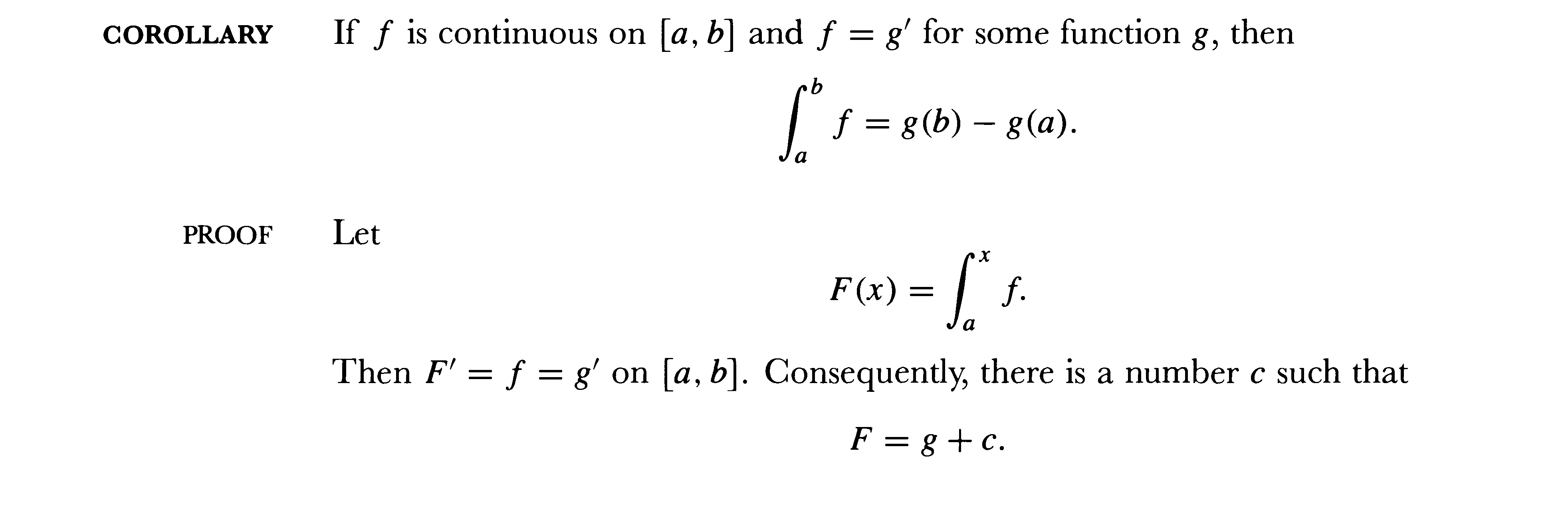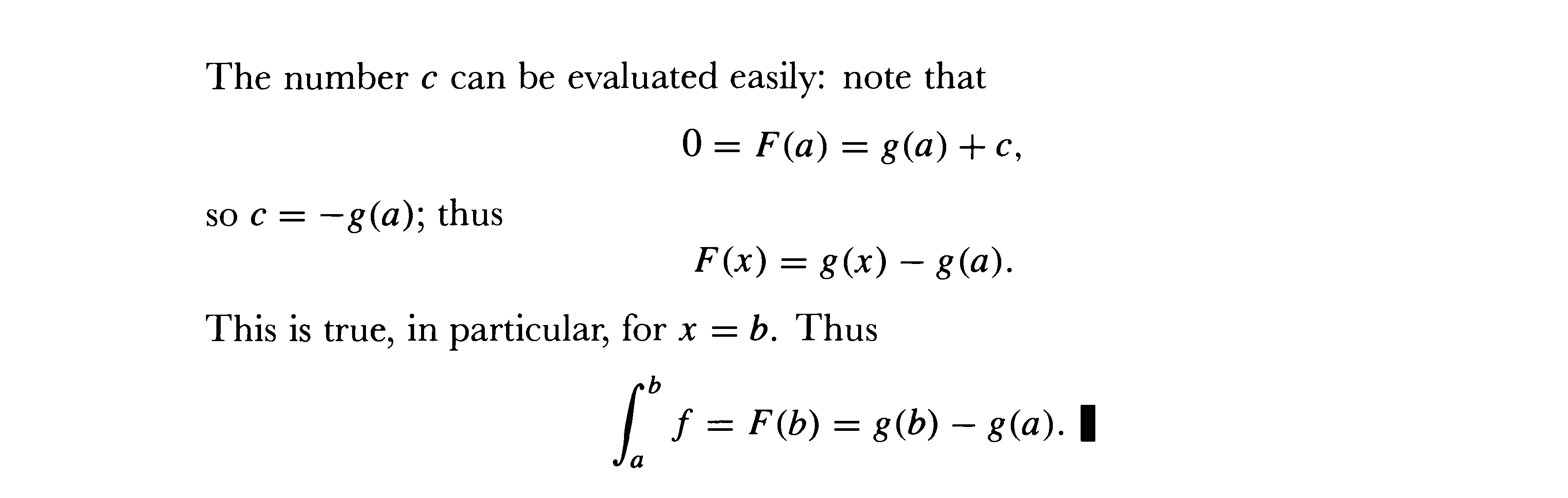Theorem: If f  is continuous on [a,b] then f is integrable on [a,b].

Proof [From Spivak, Calculus, Ch 14]